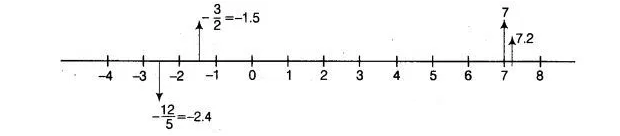# Represent the following numbers`
Question:

Represent the following numbers on the number line $7,7.2,-3 / 2$ and $-12 / 5$.

Solution:

Firstly, we draw a number line whose mid-point is 0 . Marks a positive numbers on right hand side of 0 and negative numbers on left hand side of 0 .(i) Number 7 is a positive number. So we mark a number 7 on the right hand side of 0, which is a 7 units distance from zero.

(ii) Number 7.2 is a positive number. So, we mark a number 7.2 on the right hand side of 0, which is a 7.2 units distance from zero.

(iii) Number -3/2 or -1.5 is a negative number. So, we mark a number 1.5 on the left hand side of 0, which is a 1.5 units distance from zero.

(iv) Number – 12/5 or -2.4 is a negative number. So, we mark a number 2.4 on the left hand side of 0, which is a 2.4 units distance from zero.